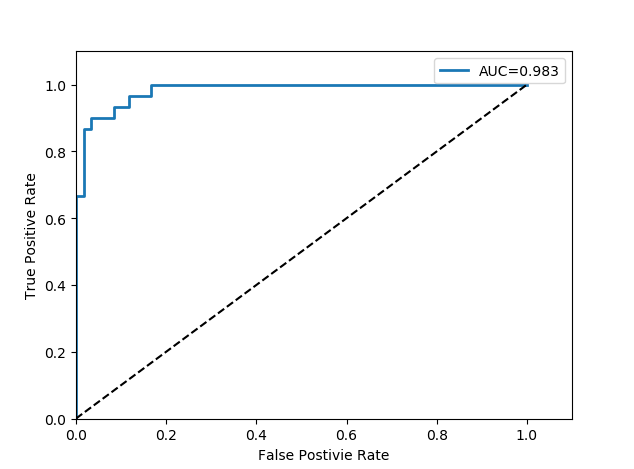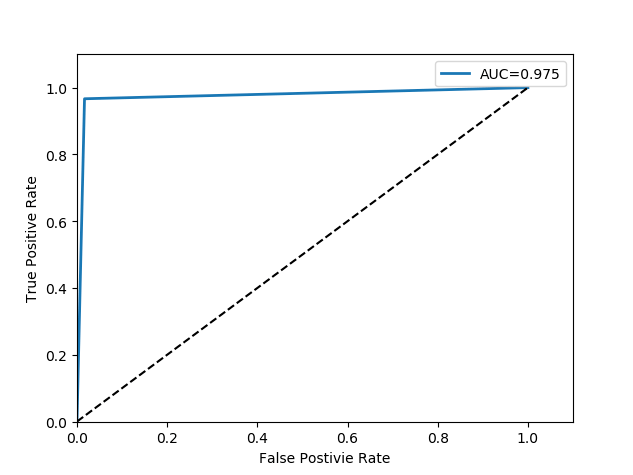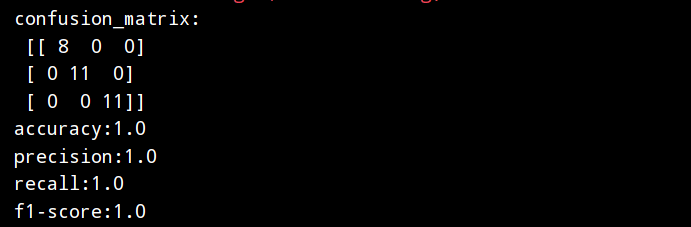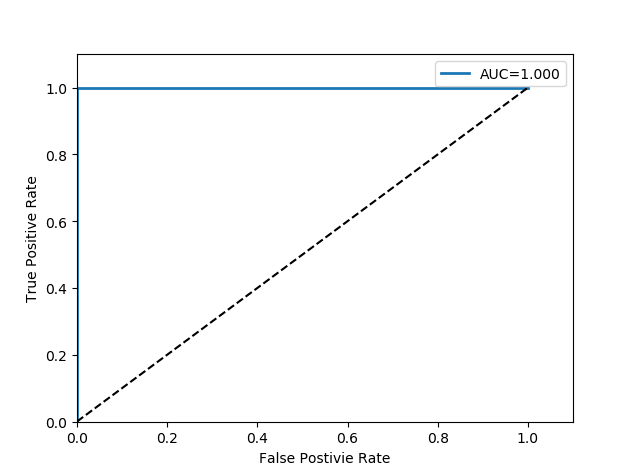# 机器学习实战︱基于线性回归、决策树和SVM进行鸢尾花分类01

fromsklearn.linear_model importLogisticRegression

fromsklearn.model_selection importtrain_test_split

importnumpy asnp

fromsklearn.preprocessing importlabel_binarize

fromsklearn.metrics importconfusion_matrix, precision_score, accuracy_score,recall_score, f1_score, roc_auc_score, \

roc_curve

importmatplotlib.pyplot asplt

# 加载数据集

X = iris_dataset.data

y = iris_dataset.target

# 将数据划分为训练集和测试集

X_train, X_test, y_train, y_test = train_test_split(X, y, test_size= 0.2)

returnX_train, X_test, y_train, y_test

# 训练Logistic模性

deftrainLS(x_train, y_train):

# Logistic生成和训练

clf = LogisticRegression

clf.fit(x_train, y_train)

returnclf

Logistic模型较为简单，不需要额外设置超参数即可开始训练。如代码清单 15-3所示，初始化Logistic模型并将模型在训练集上训练，返回训练好的模型。

# 测试模型

deftest(model, x_test, y_test):

# 将标签转换为one-hot形式

y_one_hot = label_binarize(y_test, np.arange( 3))

# 预测结果

y_pre = model.predict(x_test)

# 预测结果的概率

y_pre_pro = model.predict_proba(x_test)

# 混淆矩阵

con_matrix = confusion_matrix(y_test, y_pre)

print( 'confusion_matrix:\n', con_matrix)

print( 'accuracy:{}'.format(accuracy_score(y_test, y_pre)))

print( 'precision:{}'.format(precision_score(y_test, y_pre, average= 'micro')))

print( 'recall:{}'.format(recall_score(y_test, y_pre, average= 'micro')))

print( 'f1-score:{}'.format(f1_score(y_test, y_pre, average= 'micro')))

# 绘制ROC曲线

drawROC(y_one_hot, y_pre_pro)

defdrawROC(y_one_hot, y_pre_pro):

# AUC值

auc = roc_auc_score(y_one_hot, y_pre_pro, average= 'micro')

# 绘制ROC曲线

fpr, tpr, thresholds = roc_curve(y_one_hot.ravel, y_pre_pro.ravel)

plt.plot(fpr, tpr, linewidth= 2, label= 'AUC=%.3f'% auc)

plt.plot([ 0, 1], [ 0, 1], 'k--')

plt.axis([ 0, 1.1, 0, 1.1])

plt.xlabel( 'False Postivie Rate')

plt.ylabel( 'True Positive Rate')

plt.legend

plt.show

if__name__ == '__main__':

X_train, X_test, y_train, y_test = loadDataSet

model = trainLS(X_train, y_train)

test(model, X_test, y_test)Logistic是一个较为简单的模型，参数量较少，一般也用于较为简单的分类任务中，当任务更为复杂时，可以选取更为复杂的模型获得更好的效果，下面将使用不同的模型从而验证同一任务在不同模型下的表现。

02

fromsklearn importtree

# 训练决策树模性

deftrainDT(x_train, y_train):

# DT生成和训练

clf = tree.DecisionTreeClassifier(criterion= "entropy")

clf.fit(x_train, y_train)

returnclf

if__name__ == '__main__':

X_train, X_test, y_train, y_test = loadDataSet

model = trainDT(X_train, y_train)

test(model, X_test, y_test)03

# 训练SVM模性

fromsklearn importsvm

deftrainSVM(x_train, y_train):

# SVM生成和训练

clf = svm.SVC(kernel= 'rbf', probability= True)

clf.fit(x_train, y_train)

returnclf

if__name__ == '__main__':

X_train, X_test, y_train, y_test = loadDataSet

model = trainSVM(X_train, y_train)

test(model, X_test, y_test)04

《Python机器学习实战-微课视频版》

ISBN：978-7-302-57641-9

05

• 鲲鹏架构入门与实战︱鲲鹏应用迁移（附代码）

• 《机器学习》实验指导书（附实验参考+代码）

• Python爬虫综合实战 │ 创建云起书院爬虫（附代码）

• Python爬虫实战 │ Email提醒（附代码）

• Python深度学习 │一文掌握卷积神经网络

• Python边做边学︱商品列表信息爬取（附代码）

• P ython爬虫实战│状态521网页的爬取

• Python爬虫实战│爬取天气数据的实例详解（附源码）

• Python实训：用贪婪算法分析业务员路径问题｜附源码